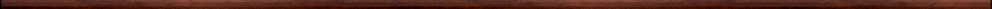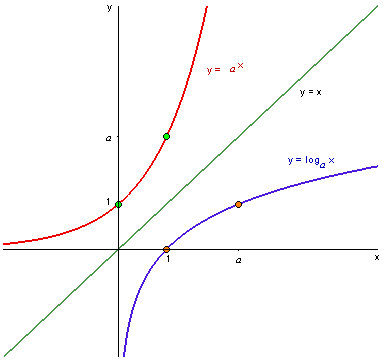Maple worksheets on exponential and logarithm functionsPrecalculus topics:

They are all compatible with Classic Worksheet Maple 10.Exponential functions and the number e - exp.mws

• Building up the domain of the exponential function f(x)=2^x ... rules for exponents.
• An introduction to the mathematical constant e via compound interest.
• Two descriptions of e.
• e is irrational.
• The exponential function.

Logarithm functions  - log.mws

• The inverse function of f  where f(x)=2^x.
• General logarithm functions.
• Properties of logarithms.

The natural logarithm function  - nat_log.mws

• The natural logarithm function.
• Examples.

More on exponential and logarithm functions  - explog.mws

• Solving equations which involve exponential and logarithm functions.
• Graphs of functions which involve exponential and logarithm functions .. inverse functions.

Top of page

Main index